# Tamagawa number

(diff) ← Older revision | Latest revision (diff) | Newer revision → (diff)

The volume of a homogeneous space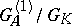associated with the group of adèles (cf. Adèle) of a connected linear algebraic groupdefined over a global fieldwith respect to the Tamagawa measure. Here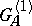is the subgroup of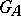consisting of those adèles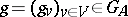for whichfor any characterofwhich is defined over(the product is taken with respect to all valuationsin the setof normalized valuations of). The finiteness of the Tamagawa number follows from reduction theory (see ).

When describing the values of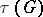it is convenient to distinguish the cases of unipotent groups, algebraic tori and semi-simple groups. For unipotent groups the Tamagawa number is always equal to 1. Ifis an algebraic-torus, thenwhereand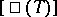are the order of the one-dimensional Galois cohomology group of the module of rational characters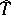of the torusand the order of its Shafarevich–Tate group, respectively. On the basis of this formula an example was constructed of a torus for which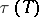is not an integer . The determination of the Tamagawa number of a semi-simple group over a number field can be reduced to the case of a simply-connected group : Letbe a semi-simple-group, let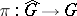be the universal covering which is defined over, let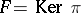be the fundamental group of, and let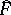be its character group; then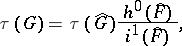where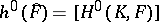, andis the order of the kernel of the canonical mapping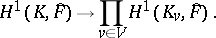It is the conjectured that for all simply-connected groups the Tamagawa number is equal to 1 (the Weil conjecture). This was proved for most types of simple groups over number fields (, , ), and also for Chevalley groups over number fields (see ) and over global function fields .

How to Cite This Entry:
Tamagawa number. Encyclopedia of Mathematics. URL: http://encyclopediaofmath.org/index.php?title=Tamagawa_number&oldid=13490
This article was adapted from an original article by A.S. Rapinchuk (originator), which appeared in Encyclopedia of Mathematics - ISBN 1402006098. See original article